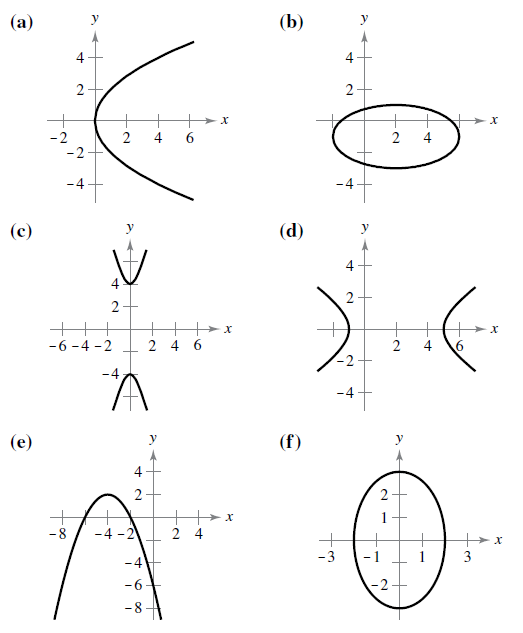Chapter 10.1, Problem 2E

Chapter
Section
Textbook Problem

# Matching In Exercises 5-10, match the equation with its graph. [The graphs are labeled (a), (b), (c), (d), (e). and (f).]( x + 4 ) 2 = − 2 ( y − 2 )

To determine
The correct match of the provided equation (x+4)2= 2(y2) with its correct graph.

Explanation

Given: The equation (x+4)2= 2(y2) and the graph of equations are,

Calculation: Consider the equation (x+4)2= 2(y2). Since the given equation is of the type (xh)2=4p(yk) which is same as the standard form of parabola with vertex at (h,k) and focal length |p|

To determine

To Graph: The equation (x2)216+(y+1)24=1

Given:### Still sussing out bartleby?

Check out a sample textbook solution.

See a sample solution

#### The Solution to Your Study Problems

Bartleby provides explanations to thousands of textbook problems written by our experts, many with advanced degrees!

Get Started

#### Find more solutions based on key concepts